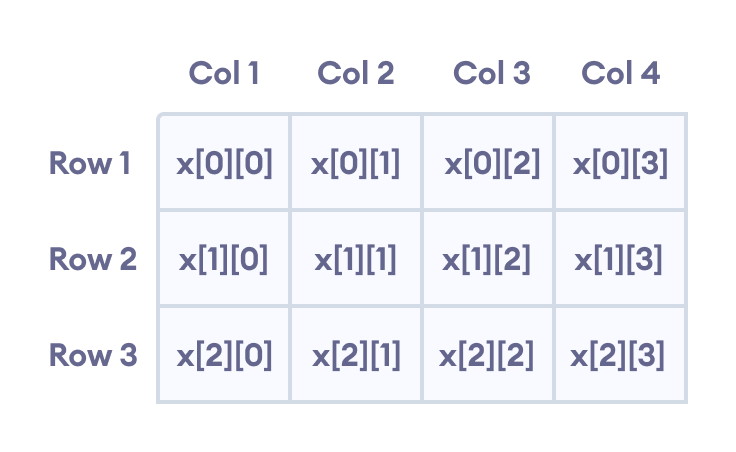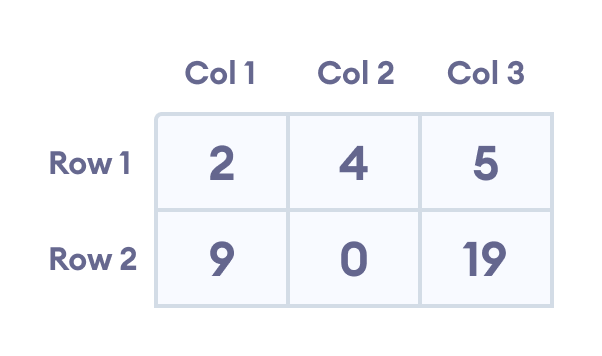Hits: 4

# C++ Multidimensional Arrays

#### In this tutorial, we’ll learn about multi-dimensional arrays in C++. More specifically, how to declare them, access them, and use them efficiently in our program.

In C++, we can create an array of an array, known as a multidimensional array. For example:
``int x;``

Here, x is a two-dimensional array. It can hold a maximum of 12 elements.

We can think of this array as a table with 3 rows and each row has 4 columns as shown below.Elements in two-dimensional array in C++ Programming

Three-dimensional arrays also work in a similar way. For example:

``float x;``

This array x can hold a maximum of 24 elements.

We can find out the total number of elements in the array simply by multiplying its dimensions:

``2 x 4 x 3 = 24``

## Multidimensional Array Initialization

Like a normal array, we can initialize a multidimensional array in more than one way.

### 1. Initialization of two-dimensional array

``int test = {2, 4, 5, 9, 0, 19};``

The above method is not preferred. A better way to initialize this array with the same array elements is given below:

``int  test = { {2, 4, 5}, {9, 0, 19}};``

This array has 2 rows and 3 columns, which is why we have two rows of elements with 3 elements each.Initializing a two-dimensional array in C++

### 2. Initialization of three-dimensional array

``````int test = {3, 4, 2, 3, 0, -3, 9, 11, 23, 12, 23,
2, 13, 4, 56, 3, 5, 9, 3, 5, 5, 1, 4, 9};``````

This is not a good way of initializing a three-dimensional array. A better way to initialize this array is:

``````int test = {
{ {3, 4, 2, 3}, {0, -3, 9, 11}, {23, 12, 23, 2} },
{ {13, 4, 56, 3}, {5, 9, 3, 5}, {5, 1, 4, 9} }
};``````

## Example 1: Two Dimensional Array

``````// C++ Program to display all elements
// of an initialised two dimensional array

#include <iostream>
using namespace std;

int main(){
int test = {{2, -5},
{4, 0},
{9, 1}};

// use of nested for loop
// access rows of the array
for (int i = 0; i < 3; ++i) {

// access columns of the array
for (int j = 0; j < 2; ++j) {
cout << "test[" << i << "][" << j << "] = " << test[i][j] << endl;
}
}

return 0;
}``````

Output

```test = 2
test = -5
test = 4
test = 0
test = 9
test = 1```

In the above example, we have initialized a two-dimensional `int` array named test that has 3 “rows” and 2 “columns”.

Here, we have used the nested `for` loop to display the array elements.

• the outer loop from `i = 0` to `i = 2` access the rows of the array
• the inner loop from `j = 0` to `j = 1` access the columns of the array

## Example 2: Taking Input for Two Dimensional Array

``````
#include <iostream>
using namespace std;

int main(){
int numbers;

cout << "Enter 6 numbers: " << endl;

// Storing user input in the array
for (int i = 0; i < 2; ++i) {
for (int j = 0; j < 3; ++j) {
cin >> numbers[i][j];
}
}

cout << "The numbers are: " << endl;

//  Printing array elements
for (int i = 0; i < 2; ++i) {
for (int j = 0; j < 3; ++j) {
cout << "numbers[" << i << "][" << j << "]: " << numbers[i][j] << endl;
}
}

return 0;
}``````

Output

```Enter 6 numbers:
1
2
3
4
5
6
The numbers are:
numbers: 1
numbers: 2
numbers: 3
numbers: 4
numbers: 5
numbers: 6```

Here, we have used a nested `for` loop to take the input of the 2d array. Once all the input has been taken, we have used another nested `for` loop to print the array members.

## Example 3: Three Dimensional Array

``````
// C++ Program to Store value entered by user in
// three dimensional array and display it.

#include <iostream>
using namespace std;

int main(){
// This array can store upto 12 elements (2x3x2)
int test = {
{
{1, 2},
{3, 4},
{5, 6}
},
{
{7, 8},
{9, 10},
{11, 12}
}
};

// Displaying the values with proper index.
for (int i = 0; i < 2; ++i) {
for (int j = 0; j < 3; ++j) {
for (int k = 0; k < 2; ++k) {
cout << "test[" << i << "][" << j << "][" << k << "] = " << test[i][j][k] << endl;
}
}
}

return 0;
}``````

Output

```test = 1
test = 2
test = 3
test = 4
test = 5
test = 6
test = 7
test = 8
test = 9
test = 10
test = 11
test = 12```

The basic concept of printing elements of a 3d array is similar to that of a 2d array.

However, since we are manipulating 3 dimensions, we use a nested for loop with 3 total loops instead of just 2.

As we can see, the complexity of the array increases exponentially with the increase in dimensions.

# Special 95% discount

## 2000+ Applied Machine Learning & Data Science Recipes

### Portfolio Projects for Aspiring Data Scientists: Tabular Text & Image Data Analytics as well as Time Series Forecasting in Python & R```
Disclaimer: The information and code presented within this recipe/tutorial is only for educational and coaching purposes for beginners and developers. Anyone can practice and apply the recipe/tutorial presented here, but the reader is taking full responsibility for his/her actions. The author (content curator) of this recipe (code / program) has made every effort to ensure the accuracy of the information was correct at time of publication. The author (content curator) does not assume and hereby disclaims any liability to any party for any loss, damage, or disruption caused by errors or omissions, whether such errors or omissions result from accident, negligence, or any other cause. The information presented here could also be found in public knowledge domains.```